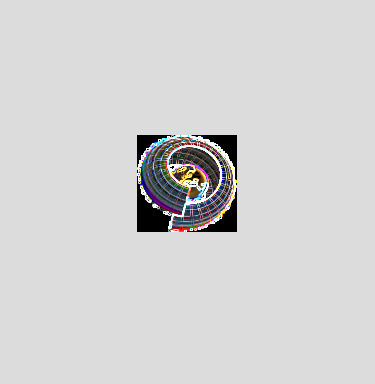# DMelt:Image/2 Image Manipulation with ImageJ

Limitted access. First login to DataMelt if you are a full DataMelt member. Then login to HandWiki as a user.

# Introduction

The example below shows how to load an image and apply a filter. It also show how to access its internals. Look at the complete API of theIJ package.

from ij import *
from ij.process import *
from ij.measure import *

file="image.png"
# we open the file manually. Generate  ImagePlus object
imp = IJ.openImage(file)
print dir(imp) # check image manipulation methods

# display it
imp.show()
print imp.getWidth()

# do manipulations with the clone
imp.getProcessor().setThreshold(174, 174, ImageProcessor.NO_LUT_UPDATE)
IJ.run(imp,"Watershed", "")
imp.show()

# get all the pixels
pix = imp.getProcessor().getPixels()

Next we can perform a detailed analysis of images. For example, one can create a histogram:

from ij import *
imp = IJ.openImage("https://datamelt.org/data_local/img/dmelt1t.png")
IJ.run(imp, "Histogram", "")
stats = imp.getStatistics()
print stats.histogram

One can extract data from image and perform manipulation. Then a new modified array can be used to build a new image. Look at the example:

## Edge detection of images

In this example, let's detect edges of the image of DMelt logo. Below we show 2 examples: one reads this logo using the URL, and the second example reads this logo from local file.

The output of these scripts is below:Note that it makes sense to convert the image into back-white. Also, one can swap the colors.

Below we will consider other topics, such as

• Creating an image from a text file
• Obtain/View histogram and measurements from an image
• Removing bleeding from one channel to another
• Subtract the minimal value to an image
• Extract a specific color channel for a given time frame
• Visualize any number of TIFF stacks in a single composite multi-color image stack
• Sort all points into a chain by distance to each other
• Correct illumination in a stack: apply the illumination of one slice to all others
• Add a mouse listener to the canvas of every open image
• Add a key listener to the canvas of every open image
• Create a virtual stack from the TIF files present in a folder
• Open the slices of a very large multi-image stack file one by one, and save each as a new image file
• Apply a binary mask to every slice in an image stack